Convergence of the 2-Point Diagonally Implicit Super Class of BBDF with OffâStep Points for Solving Stiff IVPs | OMICS International
Journal of Applied & Computational Mathematics

# Convergence of the 2-Point Diagonally Implicit Super Class of BBDF with Off‐Step Points for Solving Stiff IVPs

Babangida B* and Hamisu M

Department of Mathematics and Computer Sciences, Umaru Musa Yar'adua University, Katsina, Nigeria

*Corresponding Author:
Babangida B
Department of Mathematics and Computer Sciences
Faculty of Natural and Applied Sciences
Katsina State, Nigeria
Tel: +2347067704150
E-mail: [email protected]

Received Date: October 24, 2016; Accepted Date: November 23, 2016; Published Date: November 30, 2016

Citation: Babangida B, Hamisu M (2016) Convergence of the 2-Point Diagonally Implicit Super Class of BBDF with Off-Step Points for Solving Stiff IVPs. J Appl Computat Math 5:330. doi: 10.4172/2168-9679.1000330

Copyright: © 2016 Babangida B, et al. This is an open-access article distributed under the terms of the Creative Commons Attribution License, which permits unrestricted use, distribution, and reproduction in any medium, provided the original author and source are credited.

Visit for more related articles at Journal of Applied & Computational Mathematics

#### Abstract

The 2-point Diagonally Implicit Superclass of BBDF with off-step point’s method proposed by Babangida had been studied and further established the necessary conditions for its convergence. Consistency, zero stability and order of the method are discussed.

#### Keywords

Off-step; Diagonally implicit 2-point SBBDF; Stiff ODEs; Zero stability; Consistency conditions; Convergence order

#### Introduction

Consider the following system of first order n-dimensional system of stiff IVPs of the form: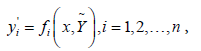(1)

with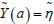, in the interval a ≤ x ≤ b, where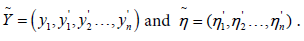System (1), which arise frequently in the study of electrical circuits, vibrations, chemical reactions, kinetics, automatic control and combustion, theory of fluid mechanics etc., is said to be stiff if its solution contains components with both slowly and rapidly decaying rates, due to large difference of time scales exhibited by the system [1,2]. Dahlquist  defines stiffness as systems containing very fast components as well as very slow components. It is difficult to develop suitable methods for stiff problems. However, considerable research efforts have been made by researchers, such as Suleiman [1,2], Musa [4-8], Abasi [9,10], Ibrahim [11,12], Zawawi  and Cash , to develop suitable methods for stiff ODEs. This paper discussed the order and convergence of diagonally implicit 2-point superclass of BBDF with off step points for solving (1) developed by Babangida  and it is as follows: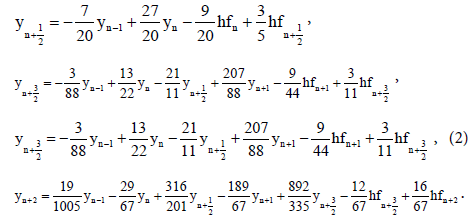The derivation of the method in details can be found in Babangida .

#### Order of the Method

This section derives the order of the method (2). Method (2) can be rewritten in matrix form as follows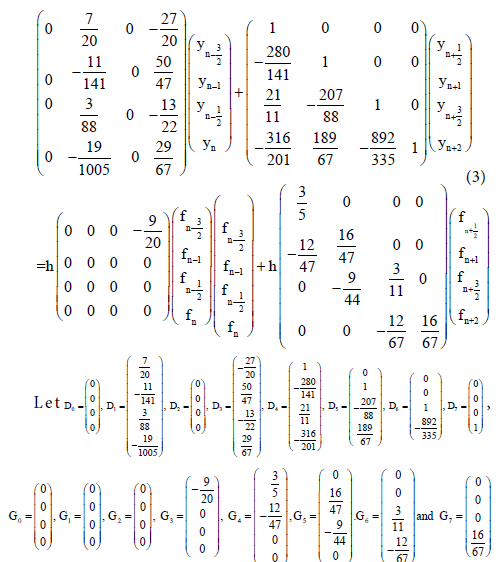Definition 2.1: The order of the block method (2) and its associated linear operator L is given by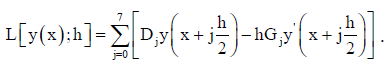(4)

Expanding the functions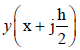and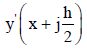as Taylor series around x and substitute in (4) gives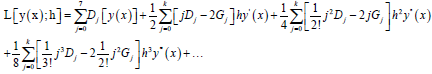(5)

Definition 2.2: The difference operator (5) and the associated method (2) is considered of order p if E0=E1=E2=…=Ep=0 and Ep+1 ≠ 0.

It follows that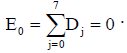(6)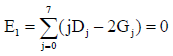(7)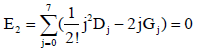(8)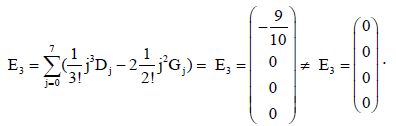(9)

It was therefore shown from (9) that method (2) is of order 2, with error constant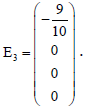#### Convergence of the Method

An acceptable linear multistep method (LMM) must be Convergence. This section shows the convergence of the method (2). Consistency and zero stability are the necessary and sufficient conditions for the convergence of any numerical method. We start by presenting the following definitions related to the convergence of (2) taken from Musa  as follows:

Definition 3.1 (Linear multistep method)

A general k-step linear multistep method is defined as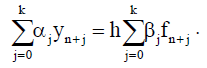(10)

Where αj and ßj are constants and αk ≠ 0.α0 and ß0 cannot both be zero at the same time. For any k step method, αk is normalized to 1.

Definition 3.2 (Linear difference operator)

The linear operator L associated LLM (10) is defined by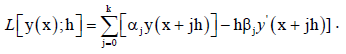(11)

Where y(x) is an arbitrary test function and it is continuously differentiable on [a, b]. Expanding y(x + jh) and ý(x+jh) as Taylor series about x, and collecting common terms yields.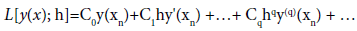(12)

Where Cq are constants given by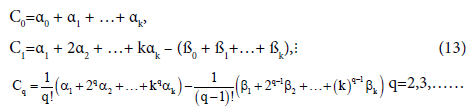Definition 3.3 (Consistency)

The LMM (10) is said to be consistent if its order p ≥ 1.

It also follows from (13) that the LMM (10) is consistent if and only if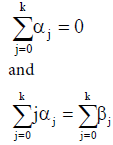(14)

Definition 3.4 (Zero stability)

A Linear Multistep Method (10) is said to be zero stable if no root of its first characteristics polynomial has modulus greater than one and that any root with modulus one is simple.

Consistency of the method

In section 2, it has shown that the 2-point DISBBDF with off-step points proposed by Babangida  is of order 2, which satisfies the consistency conditions given in definition 3.3. It now remains to show that the method is consistent.

The method (2) is consistent if and only if the following conditions are satisfied: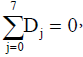(15)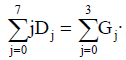(16)

Where Dj's and Gj's are previously defined in section 2.

Equation (15) then becomes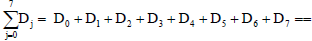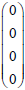,        (17)

Hence condition (15) is therefore met.

Equation (16) also becomes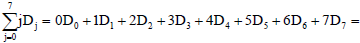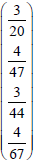,            (18)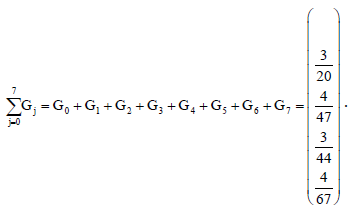(19)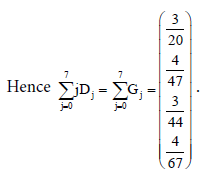(20)

Thus, the second conditions in (18) and (19) are also satisfied.

The consistency conditions are therefore met. Hence, method (2) is consistent.

#### Zero Stability

It now remains to show that the method is zero stable. The stability polynomial of the method (2) is given by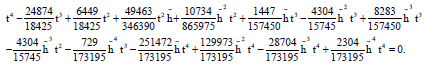(21)

For zero stability, we set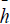=0 in (21). Hence we have: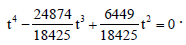(22)

Solving equation (22) for t gives the following roots:

t=0, t=0, t=0.350014 and t=1.          (23)

From the definition 3.4, method (2) is zero-stable. Since the method (2) is both consistent and zero stable, it thus method (2) converges.

#### Conclusion

The 2-point Diagonally Implicit Superclass of BBDF with off-step points method proposed by Babangida had been studied. The necessary conditions for the convergence of method is discussed. The method is zero stable and consistent. It is therefore conclude the method converges.

#### References

Select your language of interest to view the total content in your interested language

### Article Usage

• Total views: 8871
• [From(publication date):
October-2016 - Dec 07, 2019]
• Breakdown by view type
• HTML page views : 8782Can't read the image? click here to refresh